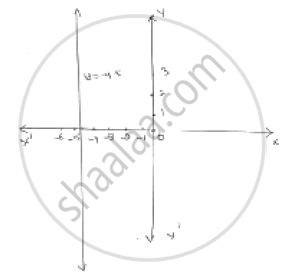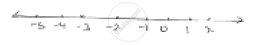# Give the Geometric Representation of the Following Equation (A) on the Number Line (B) on the Cartesian Plane : 2x + 9 = 0 - Mathematics

Give the geometric representation  of the following equation
(a) on the number line    (b) on the Cartesian plane :   2x + 9 = 0

#### Solution2x  + 9  = 0

2x = -9

 x = (-9) / 2  = - 4  . 5

Point A represents  - 4  . 5 on number line
On Cartesian plane, equation represents all points on  y  - axis for which x = - 4.5

Concept: Equations of Lines Parallel to the X-axis and Y-axis
Is there an error in this question or solution?

#### APPEARS IN

RD Sharma Mathematics for Class 9
Chapter 7 Linear Equations in Two Variables
Exercise 7.4 | Q 1.4

Share